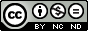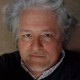## The “Standard Model”, Part I: Natural Law, Economics, Measurement, and Capital

In the late 18th and early 19th centuries, scientists took Newton’s successful study of gravitation and the laws of motion as a model for the conduct of any other field of investigation that would purport to be a science. Heilbron (1993) documents how the “Standard Model” evolved and eventually informed the quantitative study of areas of physical nature that had previously been studied only qualitatively, such as cohesion, affinity, heat, light, electricity, and magnetism. Referred to as the “six imponderables,” scientists were widely influenced in experimental practice by the idea that satisfactory understandings of these fundamental forces would be obtained only when they could be treated mathematically in a manner analogous, for instance, with the relations of force, mass, and acceleration in Newton’s Second Law of Motion.

The basic concept is that each parameter in the model has to be measurable independently of the other two, and that any combination of two parameters has to predict the third.  These relationships are demonstrably causal, not just unexplained associations. So force has to be the product of mass and acceleration; mass has to be force divided by acceleration; and acceleration has to be force divided by mass.

The ideal of a mathematical model incorporating these kinds of relations not only guided much of 19th century science, the effects of acceleration and force on mass were a vital consideration for Einstein in his formulation of the relation of mass and energy relative to the speed of light, with the result that energy is now separated from mass in the context of relativity theory (Jammer, 1999, pp. 41-42). He realized that, in the same way humans experience nothing unpleasant or destructive as body mass (or, as is now held, its energy) increases when accelerated to the relatively high speeds of trains, so, too, might we experience similar changes in the relation of mass and energy relative to the speed of light. The basic intellectual accomplishment, however, was one in a still-growing history of analogies from the Standard Model, which itself deeply indebted to the insights of Plato and Euclid in geometry and arithmetic (Fisher, 1992).

Working an independent line of research, historians of economics and econometrics have documented another extension of the Standard Model. The analogies to the new field of energetics made in the period of 1850-1880, and the use of the balance scale as a model by early economists, such as Stanley Jevons and Irving Fisher, are too widespread to ignore.  Mirowski (1988, p. 2) says that, in Walras’ first effort at formulating a mathematical expression of economic relations, he “attempted to implement a Newtonian model of market relations, postulating that ‘the price of things is in inverse ratio to the quantity offered and in direct ratio to the quantity demanded.'”

Jevons similarly studied energetics, in his case, with Michael Faraday, in the 1850s. Pareto also trained as an engineer; he made “a direct extrapolation of the path-independence of equilibrium energy states in rational mechanics and thermodynamics” to “the path-independence of the realization of utility” (Mirowski, 1988, p. 21).

The concept of equilibrium models stems from this work, and was also extensively elaborated in the analogies Jan Tinbergen was well known for drawing between economic phenomena and James Clerk Maxwell’s encapsulation of Newton’s second law. In making these analogies, Tinbergen was deliberately employing Maxwell’s own method of analogy for guiding his thinking (Boumans, 2005, p. 24).

In his 1934-35 studies with Frisch in Oslo and with Ronald Fisher in London, the Danish mathematician Georg Rasch (Andrich, 1997; Wright, 1980) made the acquaintance of a number of Tinbergen’s students, such as Tjalling Koopmans (Bjerkholt 2001, p. 9), from whom he may have heard of Tinbergen’s use of Maxwell’s method of analogy (Fisher, 2008). Rasch employs such an analogy in the presentation of his measurement model (1960, p. 115), pointing out

“…the acceleration of a body cannot be determined; the observation of it is admittedly liable to … ‘errors of measurement’, but … this admittance is paramount to defining the acceleration per se as a parameter in a probability distribution — e.g., the mean value of a Gaussian distribution — and it is such parameters, not the observed estimates, which are assumed to follow the multiplicative law [acceleration = force / mass].
Thus, in any case an actual observation can be taken as nothing more than an accidental response, as it were, of an object — a person, a solid body, etc. — to a stimulus — a test, an item, a push, etc. — taking place in accordance with a potential distribution of responses — the qualification ‘potential’ referring to experimental situations which cannot possibly be [exactly] reproduced.
In the cases considered [earlier in the book] this distribution depended on one relevant parameter only, which could be chosen such as to follow the multiplicative law.
Where this law can be applied it provides a principle of measurement on a ratio scale of both stimulus parameters and object parameters, the conceptual status of which is comparable to that of measuring mass and force. Thus, … the reading accuracy of a child … can be measured with the same kind of objectivity as we may tell its weight ….”

What Rasch provides in the models that incorporate this structure is a portable way of applying Maxwell’s method of analogy from the Standard Model. Data fitting a Rasch model show a pattern of associations suggesting that richer causal explanatory processes may be at work, but model fit alone cannot, of course, provide a construct theory in and of itself (Burdick, Stone, & Stenner, 2006; Wright, 1994). This echoes Tinbergen’s repeated emphasis on the difference between the mathematical model and the substantive meaning of the relationships it represents.

It also shows appreciation for the reason why Ludwig Boltzmann was so enamored of Maxwell’s method of analogy. As Boumans (1993, p. 136; also see Boumans, 2005, p. 28), “it allowed him to continue to develop mechanical explanations without having to assert, for example, that a gas ‘really’ consists of molecules that ‘really’ interact with one another according to a particular force law. If a scientific theory is only an image or a picture of nature, one need not worry about developing ‘the only true theory,’ and one can be content to portray the phenomena as simply and clearly as possible.” Rasch (1980, pp. 37-38) similarly held that a model is meant to be useful, not true.

Part II continues soon with more on Rasch’s extrapolation of the Standard Model, and references cited.1.Tuning our assessment instruments to harmonize our relationships « Livingcapitalmetrics’s Blog Says: